# AP Calculus AB : Geometric understanding of graphs of continuous functions

## Example Questions

### Example Question #1 : Geometric Understanding Of Graphs Of Continuous Functions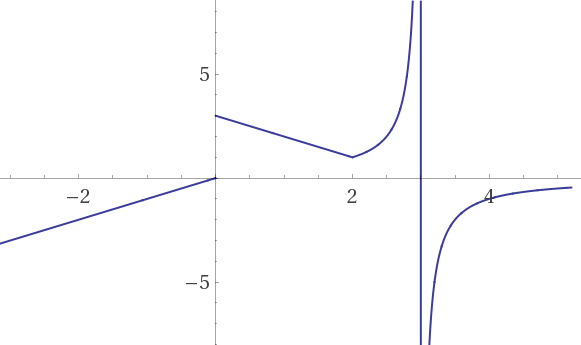is differentiable for which of the following values ofExplanation:is not differentiable atandbecause the values are discontinuities.is not differentiable atbecause that point is a corner, indicating that the one-side limits at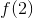are different.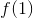is differentiable:the one side limits are the same and the point is continuous.

### Example Question #2 : Geometric Understanding Of Graphs Of Continuous Functions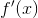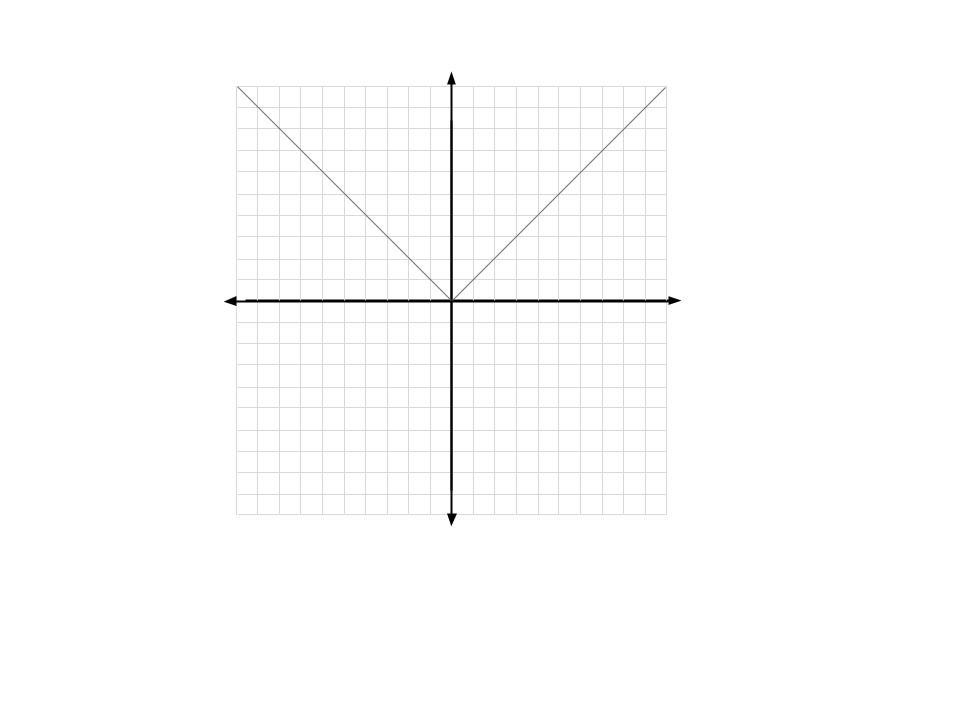Consider the graph of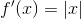above. What can we say aboutwhen?has a horizontal tangent at.is discontinuous atbecause there is a sharp turn at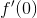.has a removable discontinuity at.

None of these are correct.

Two or more of these are correct.has a horizontal tangent at.
Note that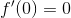, indicating that there is a horizontal tangent onat. More specifically, the derivative is the slope of the tangent line. If the slope of the tangent line is 0, then the tangent is horizontal.
The other two are incorrect because sharp turns only apply when we want to take the derivative of something. The derivative of a function at a sharp turn is undefined, meaning the graph of the derivative will be discontinuous at the sharp turn. (To see why, ask yourself if the slope atis positive 1 or negative 1?) On the other hand, integration is less picky than differentiation: We do not need a smooth function to take an integral.
In this case, to get fromto, we took an integral, so it didn't matter that there was a sharp turn at the specified point. Thus, neither function had any discontinuities.